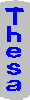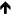Map Index Random Help## Quote: derive Fitts' Law by assuming that the relative accuracy of movement is constant; the measured time for acquiring a target is within the computed range

topics > all references > references c-d > QuoteRef: cardSK_1983 , p. 51

Group:
testing and evaluating user interfaces
Topic:
selecting with a mouse

#### Quotation Skeleton

moving the hand towards a target, can be … [refs]. … [p. 52] To make a correction [in moving to the target] takes a … Processor to decide on the correction, and one … Let X_i be the distance remaining to the … Assume that the relative accuracy of movement is … [p. 53] Hence the total movement time T_pos is … I_M log_2 (2D/S) [2.2], where I_M = -(.tau._P+.tau._C+.tau._M)/log_2 … [and D is the total distance, and S is the target width] … [p. 55] The measured correction times correspond to I_M=50 … [27 .tilde. 122] msec/bit).   Google-1   Google-2

Related Topics